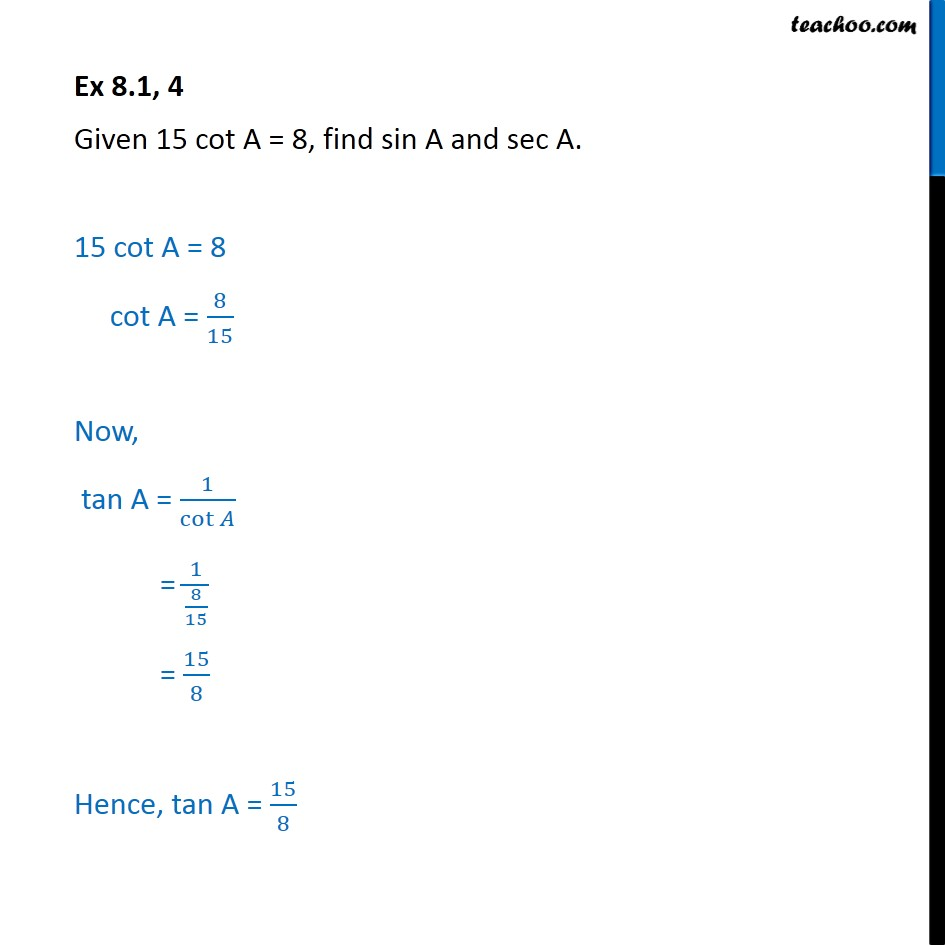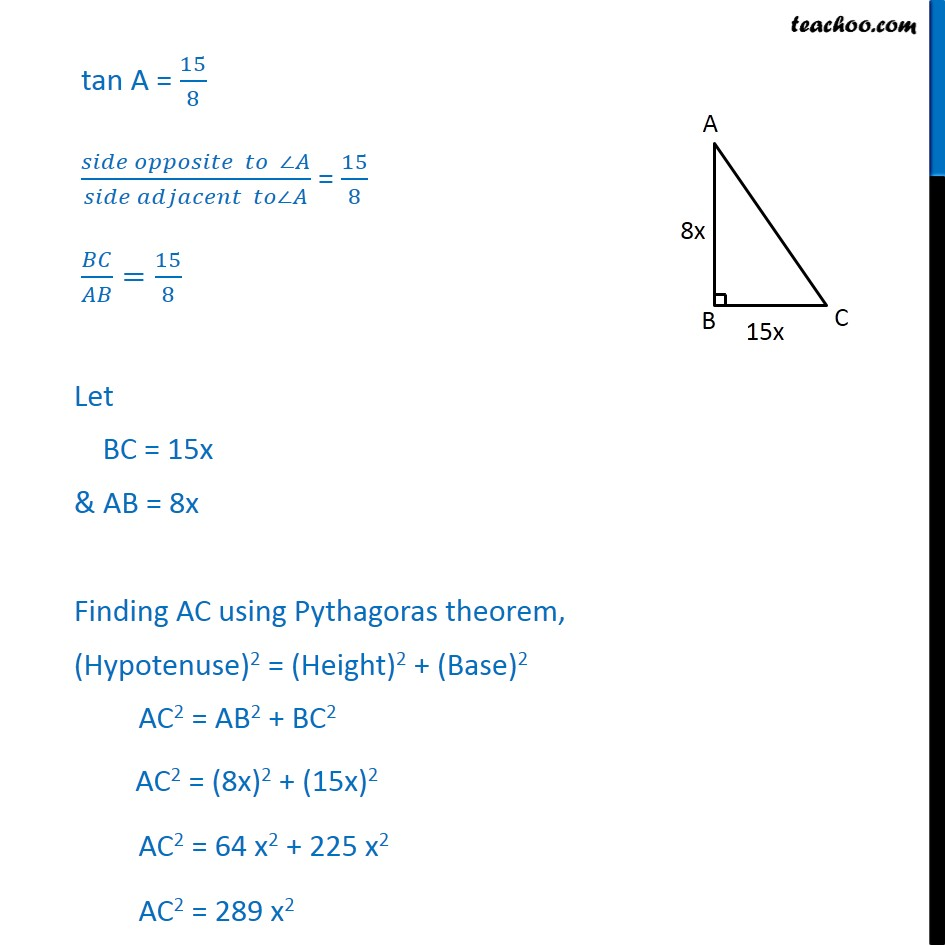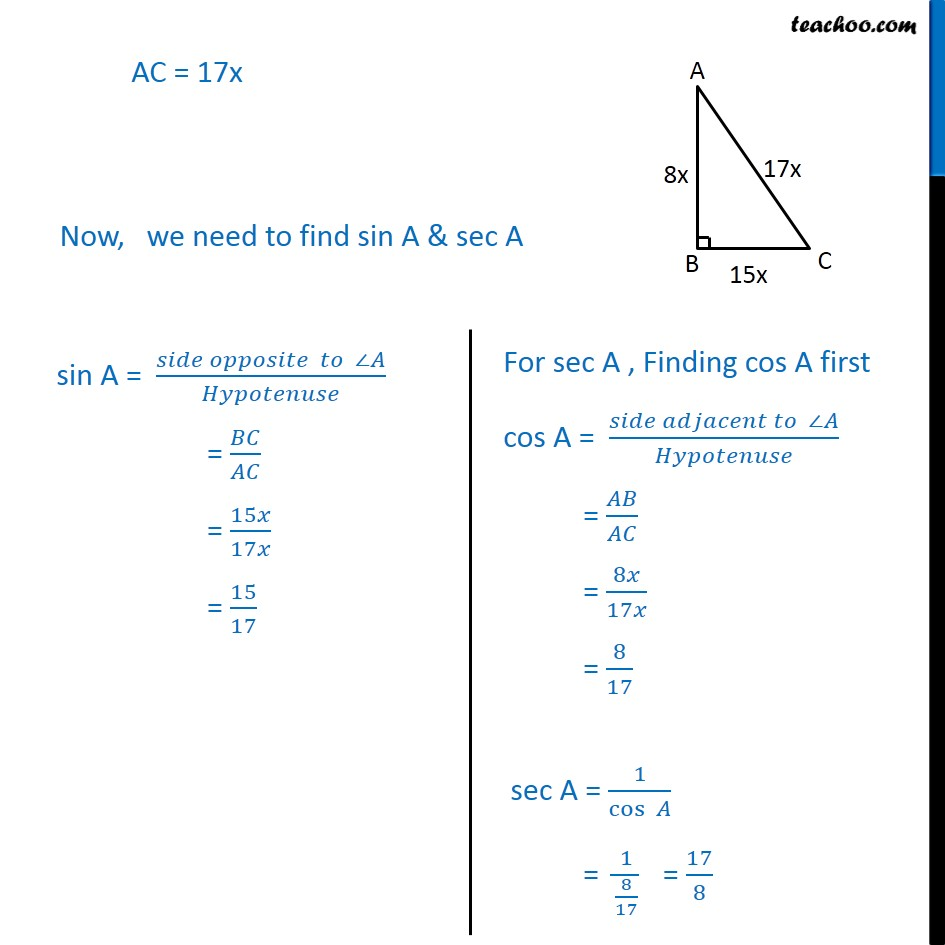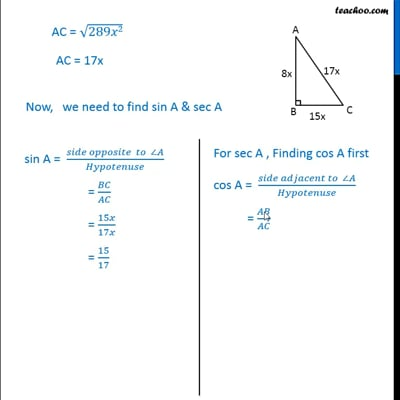Ex 8.1

Chapter 8 Class 10 Introduction to Trignometry
Serial order wiseThis video is only available for Teachoo black users

Maths Crash Course - Live lectures + all videos + Real time Doubt solving!

### Transcript

Ex 8.1, 4 Given 15 cot A = 8, find sin A and sec A. 15 cot A = 8 cot A = 8/15 Now, tan A = 1/cot = 1/(8/15) = 15/8 Hence, tan A = 15/8 tan A = 15/8 ( )/( ) = 15/8 / =15/8 Let BC = 15x & AB = 8x Finding AC using Pythagoras theorem, (Hypotenuse)2 = (Height)2 + (Base)2 AC2 = AB2 + BC2 AC2 = (8x)2 + (15x)2 AC2 = 64 x2 + 225 x2 AC2 = 289 x2 AC = 17x Now, we need to find sin A & sec A